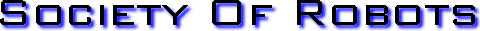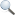Search Here MISC  Parts List  Robot Forum  Chat  Member Pages  Axon MCU  Robot Books  Shop  Contact HARDWARE  Actuators  Batteries  Electronics  Materials  Microcontrollers  Sensors SCIENCE  Robot Journals  Robot Theory  Conferences HOW TO TIME YOUR MICROCONTROLLER (WITHOUT A HARDWARE TIMER)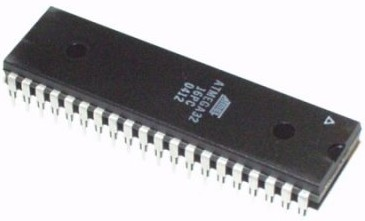Timing your Microcontroller Suppose you wanted to have your microcontroller pass some time in your software but without using a hardware timer? Hardware timers can cause problems, and every microcontroller has a limited number of them, so there are many reasons why this could be useful. I'm not saying hardware timers are bad, and in some cases its better to use them, but there are other ways to do timing that I'd like to explain. For those who are familiar with my \$50 Robot, you have probably noticed the delay_cycles function, and that its used for things such as for the servos. In this tutorial I will explain how I calculated such delay cycles and what they actually mean. Cycles A 'cycle' is the smallest amount of time it takes for your microcontroller to do 'nothing.' For example, suppose I ran this while loop on a microcontroller: ```cycles=8; void delay_cycles(unsigned long int cycles) { while(cycles > 0) cycles--; } ``` Although that while loop is effectively doing nothing, its serving another purpose of passing time. In this case 8 cycles, representing some unknown time, will pass. How long does a cycle take? Well this depends on many things. It depends on the microcontroller hardware, the frequency of the attached crystal, software settings, input voltage, fuses, etc. How do you calculate it? There are several ways, but this tutorial will demonstrate a cheap and easy way to do it experimentally. If you have an oscilloscope, you can measure exactly how long a cycle is by measuring the frequency of an output pin. Simply put this code on your microcontroller: ```loop: make digital port high delay_cycles(10); make digital port low delay_cycles(10); ``` But what if you don't have a \$2k oscilloscope? Well, there is another way . . . Attach an LED + resistor to that digital port. Then make the delay some really long time, something like this: ```loop: make digital port high delay_cycles(65500) x 10; make digital port low delay_cycles(65500) x 10; ``` What should now happen is your LED will turn on, wait a bit, then turn off, and wait a bit. Now this means that 65500*10*2 cycles occur for every flash of the LED. Next, get out your watch or Windows clock and count the number of times your LED turns on in one minute.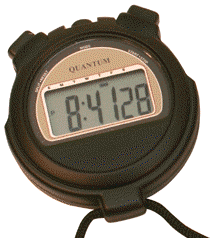Lets say you counted it flash 30 times. I will call that number 'count'. That means: 65500*10*2*count/(60 seconds)=cycles per second or if we solve that equation for our example, 655000 cycles/second But what you want is to know how many cycles it takes for a certain time period to pass, no? For example, if you wanted to control servos, you would need a square wave of 1.5ms high and 20ms low. How many cycles is 1.5ms or 20ms? calculating: 655000 cycles/second -> 655 cycles/ms 655 cycles/ms * 1.5ms = 982.5 cycles ~= 982 cycles So to get your servo to stop moving, you'd want to send a signal of 1.5ms long, or 982: ```turn servo on delay_cycles(982); turn servo off ``` Using the same equation for 1ms and 2ms, the extremes of servo motion, we calculate some more: 655 cycles/ms * 1ms = 655 cycles 655 cycles/ms * 2ms = 1310 cycles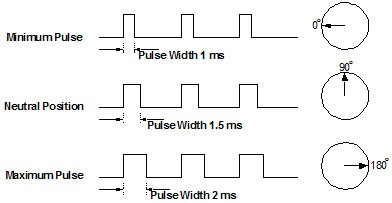The Final Equation Now suppose you wanted to create a delay of 5 seconds. Perhaps you wanted your robot to wait for some reason . . . Well, this is the equation you would use. cycles = (calculated cycles per second) * (time you want to pass) 655 cycles/ms * 5000 ms = 3275000 cycles But you aren't quite done, as a long int can only store up to 65536! Doing a delay_cycles(3275000) will not work! So calculate this: 3275000/65536 = 50 times and then program this: ```loop 50 times: delay_cycles(65535); ``` to get your 5 seconds delay. And there you have it, a method to time your microcontroller experimentally! Get Your Ad Here Has this site helped you with your robot? Give us credit - link back, and help others in the forums!Society of Robots copyright 2005-2014 forum SMF post simple machines